# 并查集(Union Find)的概念

• union(p,q)
• isConnected(p,q)

# 并查集的简单实现

## 定义接口

``````public interface UF {

int getSize();    //并查集一共有多少个元素
boolean isConnectted(int p,int q); //id为p何id为q的元素是否相连
void unionElements(int p,int q); //连接id为p和id为q的元素
}
``````

## 基本数据表示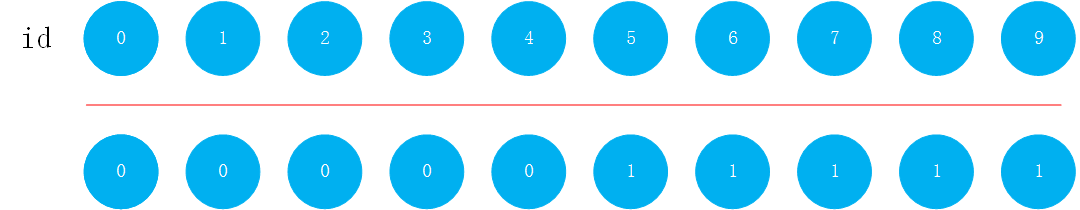# Quick Find

``````/*
QuickFind方法
unionElements(p,q)的时间复杂度为0(n)
isConnectted(p,q)的时间复杂度为0(1)
*/

public class UnionFind1 implements UF {

private int[] id;

public UnionFind1(int size){
id = new int[size];

//初始化时，每个元素对应的id都是不同的，将每个id的值设置为i对应的值
for(int i = 0 ; i < size ; i++){
id[i] = i;
}
}

@Override
public int getSize(){
return id.length;
}
//查找元素p对应的集合编号
private int find(int p){
if(p < 0 || p >= id.length)
throw new IllegalArgumentException("p is outof bound");
return id[p];
}
//查看元素p和元素q是否属于一个集合
@Override
public boolean isConnectted(int p, int q){
return find(p) == find(q);
}

``````

``````
//合并元素p和元素q所属的集合
@Override
public void unionElements(int p ,int q){
int pID = find(p);
int qID = find(q);

if(pID == qID)
return;

for(int i = 0 ; i < id.length; i++){
if(id[i] == pID){
id[i] = qID;
}
}
}
}
``````

# QuickUnion

QuickUnion的思路是把每一个元素看成树中的一个节点，并查集中的树结构是孩子指向父亲的树结构。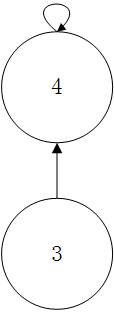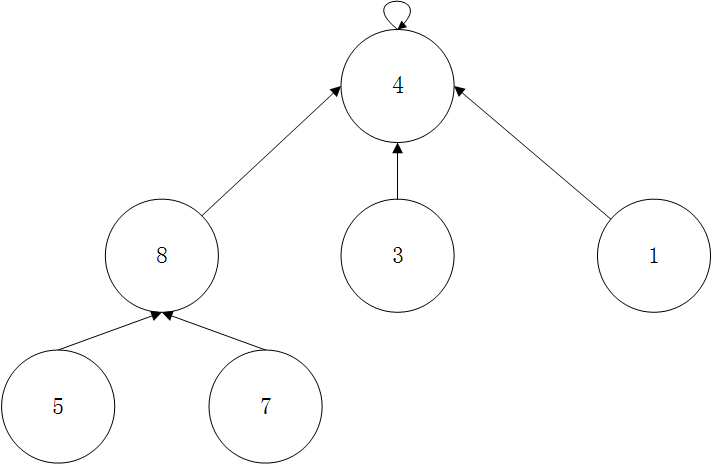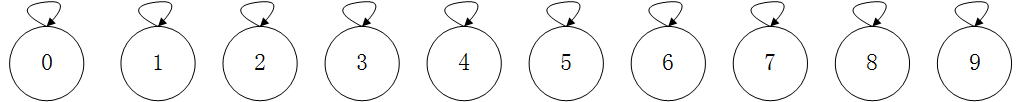0 1 2 3 4 5 6 7 8 9
0 1 2 3 4 5 6 7 8 9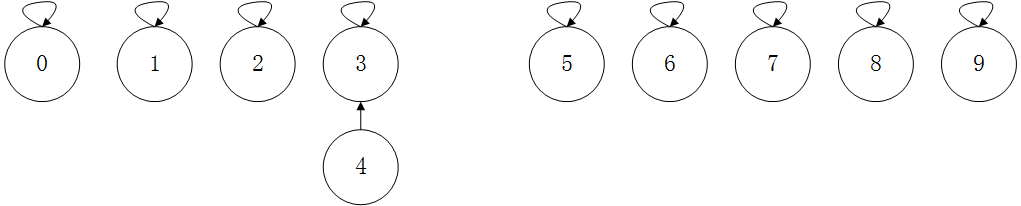0 1 2 3 4 5 6 7 8 9
0 1 2 3 3 5 6 7 8 9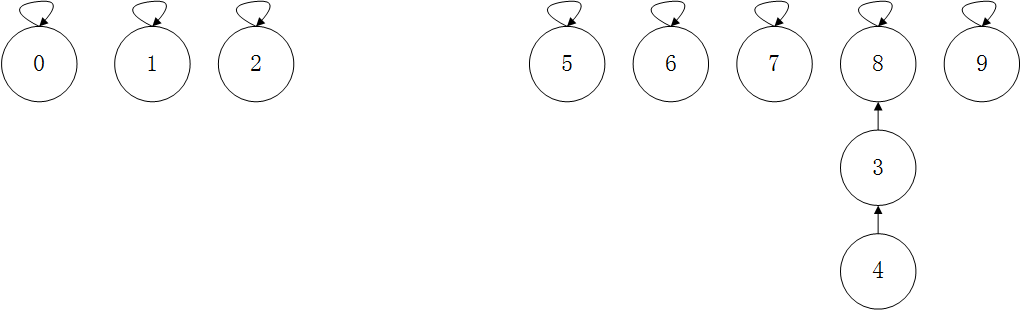0 1 2 3 4 5 6 7 8 9
0 1 2 8 3 5 6 7 8 9

0 1 2 3 4 5 6 7 8 9
0 1 2 8 3 5 6 7 8 8

``````/*
UnionFind2
QuickUnion方法
parent数组表示当前元素指向的父元素是谁
*/
public class UnionFind2 implements UF{

private int[] parent;

public UnionFind2(int size){
parent = new int[size];

for(int i = 0 ; i < size ; i++){
parent[i] = i ;
}
}

@Override
public int getSize(){
return parent.length;
}

//查找过程,查找元素p所对应的元素编号,即父节点,直到最后找到根节点
//O(h)复杂度,h为树的高度
private int find(int p){
if(p< 0 || p >= parent.length){
throw new IllegalArgumentException("p is out of bound.");
}
while (p != parent[p])
p = parent[p];
return p;
}

//查看元素p和元素q是否属于一个集合
//O(h)复杂度,h为树的高度
@Override
public boolean isConnectted(int p,int q){
return find(p) == find(q);
}

//合并元素p和元素q所属的集合
//O(h)复杂度,h为树的高度
@Override
public void unionElements(int p,int q){
int pRoot = find(p);
int qRoot = find(q);

if(pRoot == qRoot)
return;

parent[pRoot] = qRoot;
}
}
``````

# 基于size的优化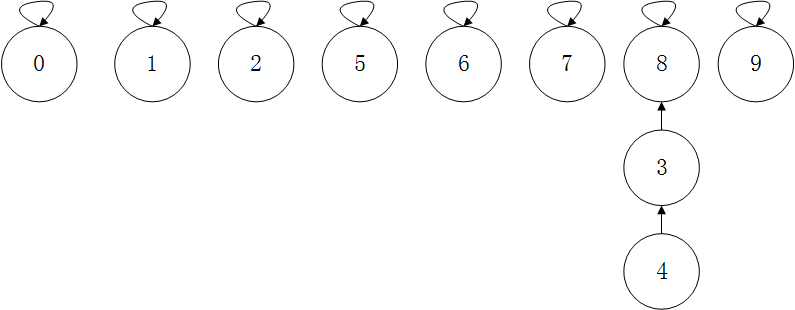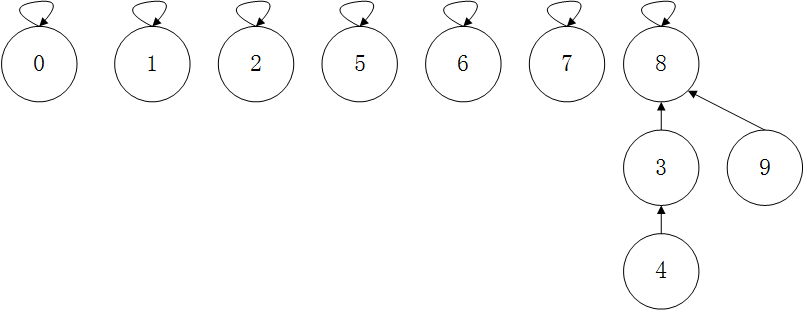``````/*
UnionFind3

QuickUnion方法
parent数组表示当前元素指向的父元素是谁
*/
public class UnionFind3 implements UF{

private int[] parent;
private int[] sz; //sz[i]表示以i为根的集合中元素的个数

public UnionFind3(int size){
parent = new int[size];
sz = new int[size];

for(int i = 0 ; i < size ; i++){
parent[i] = i ;
sz[i] = 1;
}
}

@Override
public int getSize(){
return parent.length;
}

//查找过程,查找元素p所对应的元素编号,即父节点,直到最后找到根节点
//O(h)复杂度,h为树的高度
private int find(int p){
if(p< 0 || p >= parent.length){
throw new IllegalArgumentException("p is out of bound.");
}
while (p != parent[p])
p = parent[p];
return p;
}

//查看元素p和元素q是否属于一个集合
//O(h)复杂度,h为树的高度
@Override
public boolean isConnectted(int p,int q){
return find(p) == find(q);
}

//合并元素p和元素q所属的集合
//O(h)复杂度,h为树的高度
@Override
public void unionElements(int p,int q){
int pRoot = find(p);
int qRoot = find(q);

if(pRoot == qRoot)
return;

//根据两个元素所在树的元素个数不同判断合并方向
//将元素个数少的集合合并到元素个数多的集合上
if(sz[pRoot] < sz[qRoot]){
parent[pRoot] = qRoot;
sz[qRoot] += sz[pRoot]; //qRoot为根的集合树变大了，元素数多了sz[pRoot]个
}
else{
parent[qRoot] = pRoot;
sz[pRoot] += sz[qRoot];
}

}
}
``````

# 基于rank的优化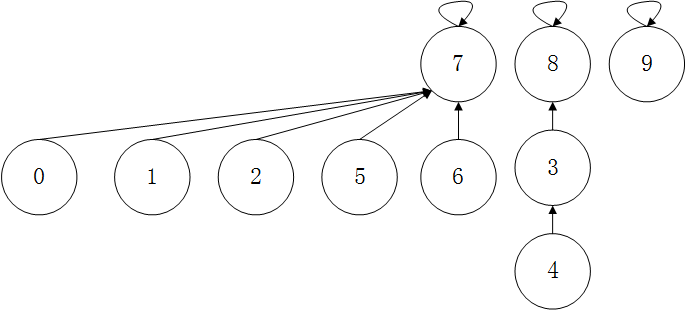0 1 2 3 4 5 6 7 8 9
7 7 7 8 3 7 7 7 8 9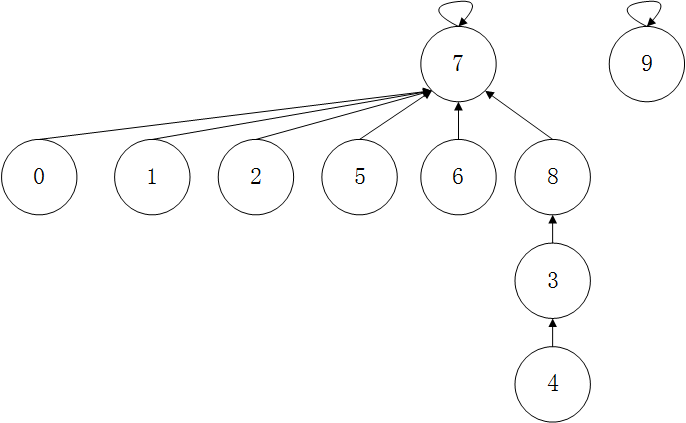但是树的深度增加了大于2的高度，树的高度高的节点指向了树的高度低的节点。

``````/*
UnionFind4

QuickUnion方法
parent数组表示当前元素指向的父元素是谁
*/
public class UnionFind4 implements UF{

private int[] parent;
private int[] rank; //rank[i]表示以i为根的集合中树的高度

public UnionFind4(int size){
parent = new int[size];
rank = new int[size];

for(int i = 0 ; i < size ; i++){
parent[i] = i ;
rank[i] = 1;
}
}

@Override
public int getSize(){
return parent.length;
}

//查找过程,查找元素p所对应的元素编号,即父节点,直到最后找到根节点
//O(h)复杂度,h为树的高度
private int find(int p){
if(p< 0 || p >= parent.length){
throw new IllegalArgumentException("p is out of bound.");
}
while (p != parent[p])
p = parent[p];
return p;
}

//查看元素p和元素q是否属于一个集合
//O(h)复杂度,h为树的高度
@Override
public boolean isConnectted(int p,int q){
return find(p) == find(q);
}

//合并元素p和元素q所属的集合
//O(h)复杂度,h为树的高度
@Override
public void unionElements(int p,int q){
int pRoot = find(p);
int qRoot = find(q);

if(pRoot == qRoot)
return;

//根据两个元素所在树的rank不同判断合并方向
//将rank低的集合合并到rank高多的集合上
if(rank[pRoot] < rank[qRoot]){
parent[pRoot] = qRoot;
}
else if(rank[qRoot] < rank[pRoot]){
parent[qRoot] = pRoot;
}
else{
parent[qRoot] = pRoot;
rank[pRoot] += 1;
}

}
}
``````

# 路径压缩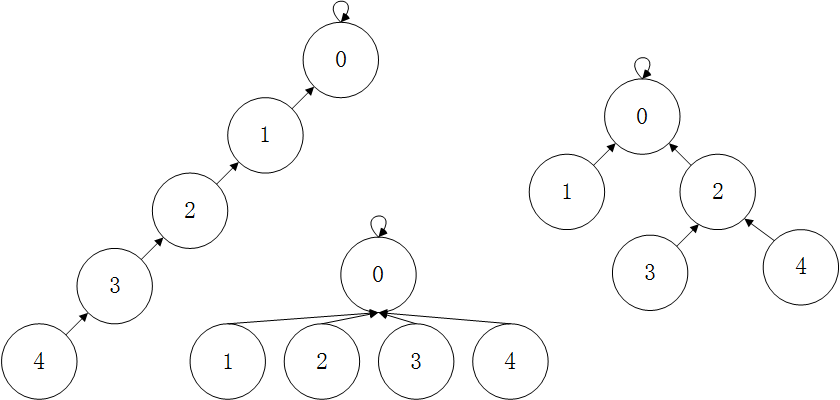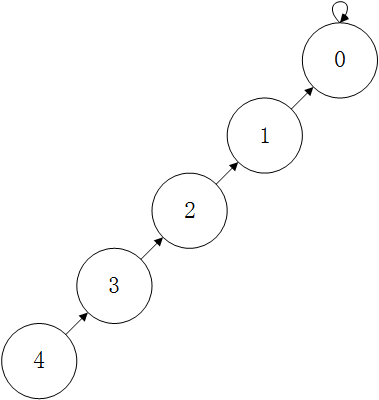0 1 2 3 4
0 0 1 2 3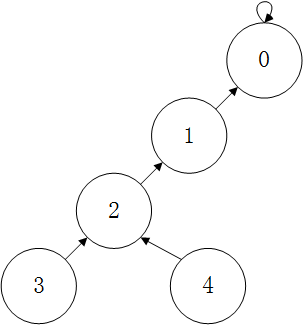0 1 2 3 4
0 0 1 2 2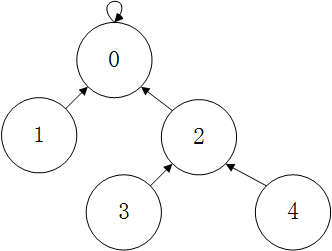0 1 2 3 4
0 0 0 2 2

``````/*
UnionFind5

QuickUnion方法
parent数组表示当前元素指向的父元素是谁
*/
public class UnionFind5 implements UF{

//其他代码相同

private int find(int p){
if(p< 0 || p >= parent.length){
throw new IllegalArgumentException("p is out of bound.");
}
while (p != parent[p]){
parent[p] = parent[parent[p]];
p = parent[p];
}
return p;
}

//..其他代码相同
``````

# 压缩为高度为2的树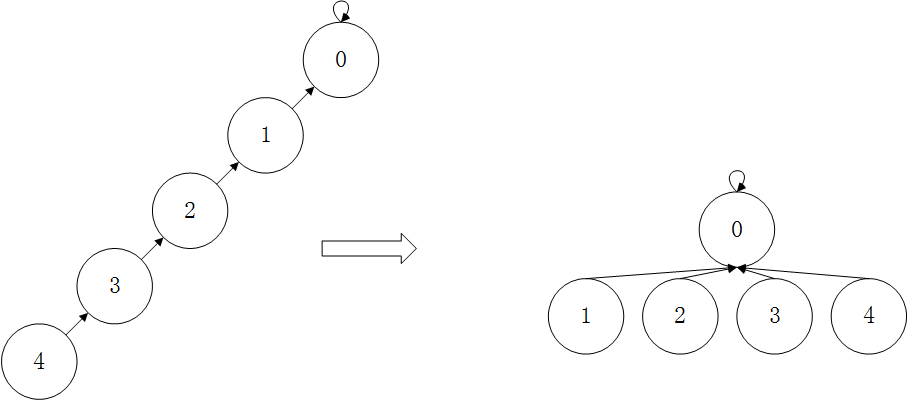0 1 2 3 4
0 0 0 0 0

``````//其他部分代码同上

private int find(int p){
if(p< 0 || p >= parent.length){
throw new IllegalArgumentException("p is out of bound.");
}
//如果当前节点不是根节点，则find它的父亲节点所对应的根节点，其实也就是p节点的根节点，然后返回parent[p],也就是整棵树的根节点
//这是从宏观语义角度进行分析
if (p != parent[p])
parent[p] = find(parent[p]);

return parent[p];
}
``````

# 并查集的时间复杂度

logn = 0 (n<=1) logn = 1 + log*(logn) (n>1)

o(log*n)的时间复杂度比log(n)还要快，近乎于o(1)